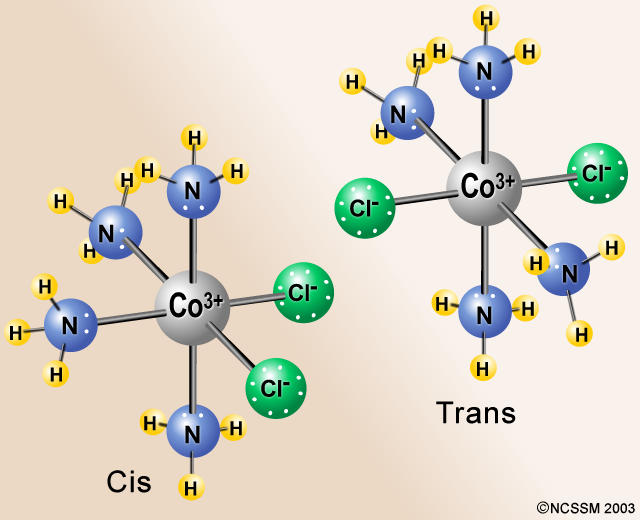# Determine whether the cis or trans isomers in [Cr(H_2O)_3Cl_3]^+?

Jan 6, 2015

[Cr(H₂O)₃Cl₃]⁺ has no cis or trans isomers.

There are six ligands, so this is an octahedral complex. You get cis and trans isomers only if the general formula is MX₄Y₂.

An example would be CoCl₂(NH₃)₄⁺.If the Cl atoms are at 90° angles to each other, the isomer is cis. If the Cl atoms are at 180° angles to each other, the isomer is trans.

For MX₃Y₃ complexes, you again get two isomers.

In the facial (fac) isomer, each set of three identical ligands occupies one face of the octahedron. Any two of these three ligands are mutually cis.If the green spheres represent Cl and the white spheres represent H₂O, the above model represents fac-[Cr(H₂O)₃Cl₃]⁺.

In the meridional (mer) isomer, each set of three identical ligands is arranged in a T-shape in a plane that includes the metal atom.